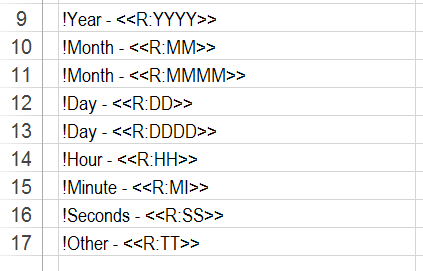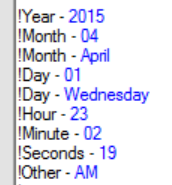Data variables are added to the templates in the format <VariableDelimiter><VariableName><VariableDelimiter>, e.g. <<IPAddress>>. The variables can be included anywhere within the template. Multiple variables may be added to a single cell.

The data variable name is prefixed and suffixed by predefined characters (defaults ‘<<‘ and ‘>>‘). These characters are defined in the Project Settings.

Date and time variables may be added the a template. The format is:

Year – <Left Variable>R:YYYY<Right Variable> = 2012, e.g. <<R:YYYY>>

Month – <Left Variable>R:MM<Right Variable> = 01, e.g. <<R:MM>>

Month – <Left Variable>R:MMMM<Right Variable> = January, e.g. <<R:MMMM>>

Day – <Left Variable>R:DD<Right Variable> = 01, e.g. <<R:DD>>

Day – <Left Variable>R:DDDD<Right Variable> = Tuesday, e.g. <<R:DDDD>>

Hour – <Left Variable>R:HH<Right Variable> = 12, e.g. <<R:HH>>

Minute – <Left Variable>R:MI<Right Variable> = 30, e.g. <<R:MI>>

Seconds – <Left Variable>R:SS<Right Variable> = 45, e.g. <<R:SS>>

Other – <Left Variable>R:TT<Right Variable> = AM or PM, e.g. <<R:TT>>

The image below shows the use of the predefined variables.The image below shows the output from the template.